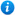# Multi-step Addition and Subtraction Problems - Year 5

Multi-step Addition and Subtraction Problems - Year 5

Year 5 maths programme of study - Number (addition and subtraction)

Pupils should be taught to:

• add and subtract whole numbers with more than 4 digits, including using formal written methods (columnar addition and subtraction)
• add and subtract numbers mentally with increasingly large numbers
• use rounding to check answers to calculations and determine, in the context of a problem, levels of accuracy
• solve addition and subtraction multi-step problems in contexts, deciding which operations and methods to use and why

In this maths teaching resource, pupils focus on solving multi-step problems as per the curriculum objectives of the Year 5 maths programme of study - Number - addition and subtraction. This lesson supports the White Rose small steps guidance for Year 5 - Autumn - Block 2 - Addition and Subtraction. Content includes:

• Animated PowerPoint lesson with class activities
• 3 differentiated worksheets with answers

'Multi-step Addition and Subtraction Problems - Year 5' is completely editable giving teachers the freedom to adapt the resource to suit their individual teaching needs.

Click on the images from the PowerPoint presentation to view the resource in more detail.

Our Price : £1.99 / 2 Credits## Get this resource as part of a bundle and save up to 35%## Year 5 Addition and Subtraction Bundle

£8.99

By continuing to use the site, you agree to the use of cookies. You can change this and find out more by following this link